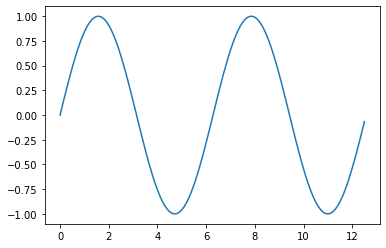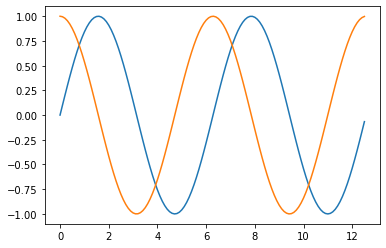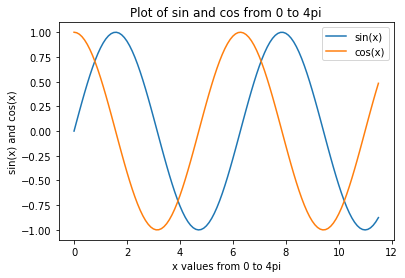Plotting is an essential skill for Engineers. Plots can reveal trends in data and outliers. Plots are a way to visually communicate results with your engineering team, supervisors and customers. In this post, we are going to plot a couple of trig functions using Python and matplotlib. Matplotlib is a plotting library that can produce line plots, bar graphs, histograms and many other types of plots using Python. Matplotlib is not included in the standard library. If you downloaded Python from python.org, you will need to install matplotlib and numpy with pip on the command line.

> pip install matplotlib
> pip install numpy


If you are using the Anaconda distribution of Python (which is the distribution of Python I recommend for undergraduate engineers) matplotlib and numpy (plus a bunch of other libraries useful for engineers) are included. If you are using Anaconda, you do not need to install any additional packages to use matplotlib.

In this post, we are going to build a couple of plots which show the trig functions sine and cosine. We'll start by importing matplotlib and numpy using the standard lines import matplotlib.pyplot as plt and import numpy as np. This means we can use the short alias plt and np when we call these two libraries. You could import numpy as wonderburger and use wonderburger.sin() to call the numpy sine function, but this would look funny to other engineers. The line import numpy as np has become a common convention and will look familiar to other engineers using Python. In case you are working in a Juypiter notebook, the %matplotlib inline command is also necessary to view the plots directly in the notebook.

In :
import matplotlib.pyplot as plt
import numpy as np
# if using a jupyter notebook
%matplotlib inline


Next we will build a set of x values from zero to 4π in increments of 0.1 radians to use in our plot. The x-values are stored in a numpy array. Numpy's arange() function has three arguments: start, stop, step. We start at zero, stop at 4π and step by 0.1 radians. Then we define a variable y as the sine of x using numpy's sin() function.

In :
x = np.arange(0,4*np.pi,0.1)   # start,stop,step
y = np.sin(x)


To create the plot, we use matplotlib's plt.plot() function. The two arguments are our numpy arrays x and y. The line plt.show() will show the finished plot.

In :
plt.plot(x,y)
plt.show()Next let's build a plot which shows two trig functions, sine and cosine. We will create the same two numpy arrays x and y as before, and add a third numpy array z which is the cosine of x.

In :
x = np.arange(0,4*np.pi,0.1)   # start,stop,step
y = np.sin(x)
z = np.cos(x)


To plot both sine and cosine on the same set of axies, we need to include two pair of x,y values in our plt.plot() arguments. The first pair is x,y. This corresponds to the sine function. The second pair is x,z. This correspons to the cosine function. If you try and only add three arguments as in plt.plot(x,y,z), your plot will not show sine and cosine on the same set of axes.

In :
plt.plot(x,y,x,z)
plt.show()Let's build one more plot, a plot which shows the sine and cosine of x and also includes axis labels, a title and a legend. We build the numpy arrays using the trig functions as before:

In :
x = np.arange(0,4*np.pi-1,0.1)   # start,stop,step
y = np.sin(x)
z = np.cos(x)


The plt.plot() call is the same as before using two pairs of x and y values. To add axis labels we will use the following methods:

matplotlib method description example
plt.xlabel() x-axis label plt.xlabel('x values from 0 to 4pi')
plt.ylabel() y-axis label plt.ylabel('sin(x) and cos(x)')
plt.title() plot title plt.title('Plot of sin and cos from 0 to 4pi')
plt.legend([ ]) legend plt.legend(['sin(x)', 'cos(x)'])

Note that plt.legend() method requires a list of strings (['string1', 'string2']), where the individual strings are enclosed with qutoes, then seperated by commas and finally inclosed in brackets to make a list. The first string in the list corresponds to the first x-y pair when we called plt.plot() , the second string in the list corresponds to the second x,y pair in the plt.plot() line.

In :
plt.plot(x,y,x,z)
plt.xlabel('x values from 0 to 4pi')  # string must be enclosed with quotes '  '
plt.ylabel('sin(x) and cos(x)')
plt.title('Plot of sin and cos from 0 to 4pi')
plt.legend(['sin(x)', 'cos(x)'])      # legend entries as seperate strings in a list
plt.show()